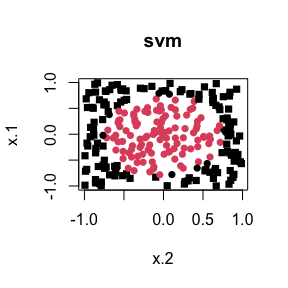# Nonparametric Regression with Bayesian Additive Regression Kernels

#### 2023-04-17

Bayesian Additive Regression Kernel (BARK) models are a flexible Bayesian nonparametric model for regression and classification problems where the unknown mean function is represented as a weighted sum of multivariate Gaussian kernel functions, $\begin{equation} f(\mathbf{x}) = \sum_j \beta_j \prod_d \exp( \lambda_d (x_d - \chi_{jd})^2) \end{equation}$ that allows nonlinearities, interactions and feature selection using Levy random fields to construct a prior on the unknown function. Each kernel is centered at location parameters $$\chi_{jd}$$ with precision parameters $$\lambda_d$$ - these precision parameters capture the importance of each of the $$d$$ dimensional predictor variables and by setting a $$\lambda_d$$ to zero may remove important variables that are not important.

## Installation

To get the latest version of {r bark}, install from github (needs compilation))

devtools::install_github("merliseclyde/bark")
library(bark)

## Example

We will illustrate feature selection in a simple simulated example from Friedman

set.seed(42)
traindata <- sim_Friedman2(200, sd=125)
testdata <- sim_Friedman2(1000, sd=0)
set.seed(42)
fit.bark.d <- bark(y ~ ., data = data.frame(traindata),
testdata= data.frame(testdata),
classification=FALSE,
selection = FALSE,
common_lambdas = FALSE,
nburn = 100,
nkeep = 250,
printevery = 10^10)

mean((fit.bark.d$yhat.test.mean-testdata$y)^2)
#>  1637.79
set.seed(42)
fit.bark.sd <- bark(y ~ ., data=data.frame(traindata),
testdata = data.frame(testdata),
classification=FALSE,
selection = TRUE,
common_lambdas = FALSE,
nburn = 100,
nkeep = 250,
printevery = 10^10)

mean((fit.bark.sd$yhat.test.mean-testdata$y)^2)
#>  1441.64

bark is similar to SVM, however it allows different kernel smoothing parameters for every dimension of the inputs $$x$$ as well as selection of inputs by allowing the kernel smoothing parameters to be zero.

The plot below shows posterior draws of the $$\lambda$$ for the simulated data.

boxplot(as.data.frame(fit.bark.d$theta.lambda))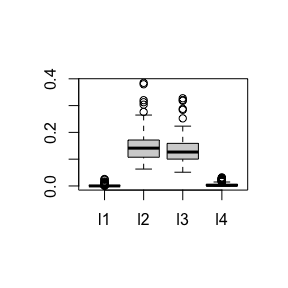boxplot(as.data.frame(fit.bark.sd$theta.lambda))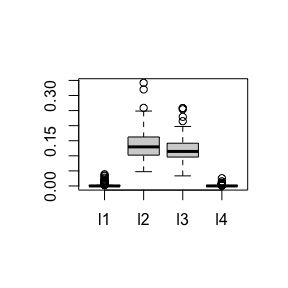The posterior distribution for $$\lambda_1$$ and $$\lambda_4$$ are concentrated at zero, which leads to $$x_1$$ and $$x_2$$ dropping from the mean function.

## Comparison

We will compare {r bark} to two other popular methods, {r BART} and {r SVM} that provide flexible models that are also non-linear in the input variables.

bart.available =  require(BART)
svm.available  =  require(e1071)
#> Loading required package: e1071

### Generate data

set.seed(42)
n = 500
circle2 = data.frame(sim_circle(n, dim = 2))
train = sample(1:n, size = floor(n/2), rep=FALSE)
plot(x.1 ~ x.2, data=circle2, col=y+1)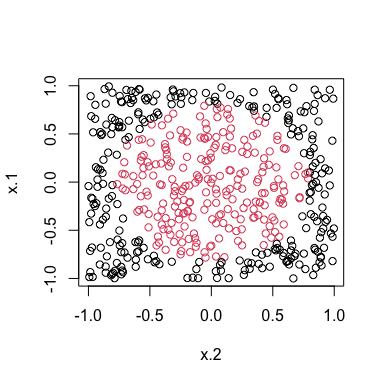circle2.bark = bark(y ~ ., data=circle2, subset=train,
testdata = circle2[-train,],
classification = TRUE,
selection = TRUE,
common_lambdas = TRUE,
nburn = 100,
nkeep = 250,
printevery = 10^10)
#Classify
#
mean((circle2.bark$yhat.test.mean > 0) != circle2[-train, "y"]) #>  0.016 ## SVM if (svm.available) { circle2.svm = svm(y ~ x.1 + x.2, data=circle2[train,], type="C") pred.svm = predict(circle2.svm, circle2[-train,]) mean(pred.svm != circle2[-train, "y"]) } #>  0.036 if (bart.available) { circle.bart = pbart(x.train = circle2[train, 1:2], y.train = circle2[train, "y"]) pred.bart = predict(circle.bart, circle2[-train, 1:2]) mean((pred.bart$prob.test.mean > .5) != circle2[-train, "y"])
}
#> *****Into main of pbart
#> *****Data:
#> data:n,p,np: 250, 2, 0
#> y1,yn: 1, 1
#> x1,x[n*p]: 0.205937, -0.132049
#> *****Number of Trees: 50
#> *****Number of Cut Points: 100 ... 100
#> *****burn and ndpost: 100, 1000
#> *****Prior:mybeta,alpha,tau: 2.000000,0.950000,0.212132
#> *****binaryOffset: -0.140835
#> *****Dirichlet:sparse,theta,omega,a,b,rho,augment: 0,0,1,0.5,1,2,0
#> *****nkeeptrain,nkeeptest,nkeeptreedraws: 1000,1000,1000
#> *****printevery: 100
#> *****skiptr,skipte,skiptreedraws: 1,1,1
#>
#> MCMC
#> done 0 (out of 1100)
#> done 100 (out of 1100)
#> done 200 (out of 1100)
#> done 300 (out of 1100)
#> done 400 (out of 1100)
#> done 500 (out of 1100)
#> done 600 (out of 1100)
#> done 700 (out of 1100)
#> done 800 (out of 1100)
#> done 900 (out of 1100)
#> done 1000 (out of 1100)
#> time: 1s
#> check counts
#> trcnt,tecnt: 1000,0
#> *****In main of C++ for bart prediction
#> number of bart draws: 1000
#> number of trees in bart sum: 50
#> number of x columns: 2
#> from x,np,p: 2, 250
#> ***using serial code
#>  0.044

Compare classification across methods.


plot(x.1 ~ x.2, data=circle2[-train,], pch = y+15,
col=(1 + (circle2.bark$yhat.test.mean > 0)), main="bark")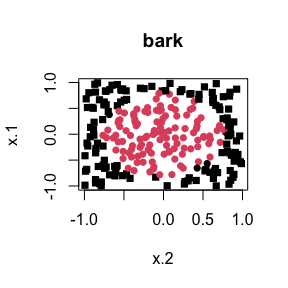if (bart.available) { plot(x.1 ~ x.2, data=circle2[-train,], pch = y+15, col= ( 1 + (pred.bart$prob.test.mean > .5)),
main="BART")
}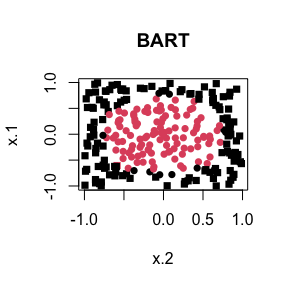if (svm.available) {
plot(x.1 ~ x.2, data=circle2[-train,], pch = y+15,
col= as.numeric(pred.svm),
main="svm")
}